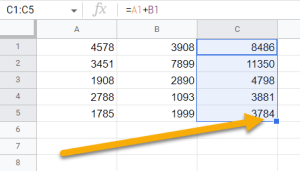To add numbers together in Google Sheets, click on the cell where you want the result, go to the Formula bar, type the numbers you wish to add together with a plus sign between them, and press Enter.

In this article, you will learn multiple methods for how to add numbers in Google Sheets. Choose the method that works best for you!

## Using the Plus Sign

This is the easiest and most straightforward method. Just follow these simple steps.

Difficulty: Beginner

Time Estimate: 5 Seconds

1. Select the cell where you want the result (B1).
2. Go to the Formula bar and type the formula (=4578+3908 in our example). Be sure to join the numbers with a plus sign to add them together.
3. Press the Enter button on your keyboard.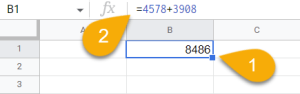Voila! The answer will display in the cell.

## Using Cell References

You can also add numbers in Google Sheets using cell references, which is useful if the data may change over time. Cell references are like variables that you can use to refer to a cell or a range of cells. Here is a step-by-step guide on how you can add using this method.

Difficulty: Beginner

Time Estimate: 5 Seconds

1. Click on the cell where you need the result to be displayed (C1).
2. In the Formula bar, type the formula using cell references (=A1+B1). Make sure the cells have numbers in them or the formula will not work.
3. Hit Enter.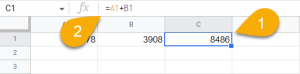It’s that simple!

## Using Formulas

As an alternate method, there are a couple formulas you can use to add numbers together.

Difficulty: Beginner

Time Estimate: 10 Seconds

### The SUM Function

In Google Sheets, you can add a range of cells with the SUM function. Let’s see how this works.

1. Select the cell where the answer should be (F1).
2. Navigate to the Formula bar and enter the formula =SUM(A1, B1, C1, D1), where A1, B1, C1, D1 are the cells with the numbers you want to add together.
3. Use your keyboard to press Enter.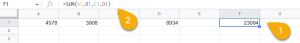Instead of entering cell references individually, you can also select a range of cells (=SUM(A1:E1)).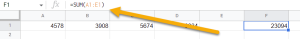This function is very similar to the SUM function. Both are extremely useful when adding together multiple cells.

1. Start by selecting the cell where you want the result (D1).
2. Go to the Formula bar and type the formula =ADD(A1, B1). In our case, A1 and B1 are cells with values.
3. Tap the Enter button on the keyboard.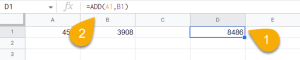Easy-peasy! Just like that, you’ve done it!

To better understand this subject, let’s take a closer look at some of the most frequently asked questions and their responses.

### Why is my SUM function not working in Google Sheets?

There are a few possible reasons why your SUM function might not be working in Google Sheets. These include:

• You have not selected the correct range of cells to sum up.
• There may be hidden cells in your selection that are preventing the SUM function from working properly.
• There may be empty cells in your selection that are preventing the SUM function from working properly.
• You may have accidentally included a cell that contains text instead of a number.

### How do I repeat a function in other cells?

To repeat a function or formula in other cells, click on the cell with the number and use the suggested autofill for the other cells.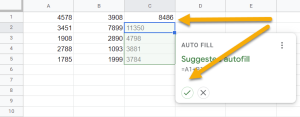As an alternative, you can drag the first cell down to achieve the same result. Be sure to drag it by the corner icon in order to copy whatever formula you may have set up in the first cell so it will be repeated in the remaining cells.